# Cosine rule

By Martin McBride, 2023-03-17
Tags: triangle cosine cosine rule sine rule solving triangles
Categories: gcse trigonometryThe cosine rule is a trigonometry formula that relates the sides and angles of a triangle. It can be used to solve a triangle if we know either:

• Two sides of the triangle, and the angle enclosed between those sides.
• Three sides but none of the angles.

For other cases, you will need to use the sine rule.

The rule applies to any triangle, not just right-angled triangles.

## Labelling the triangle

It is important to label the triangle correctly, otherwise the rule won't work! We name the angles A, B and C, and we name the sides a, b and c: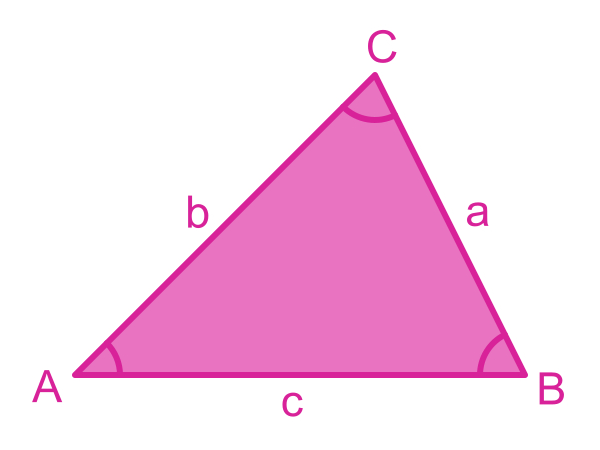The important thing to remember is that each angle is opposite the side of the same name:

• Angle A is opposite side a.
• Angle B is opposite side b.
• Angle C is opposite side c.

## The cosine rule

The cosine rule tells us that: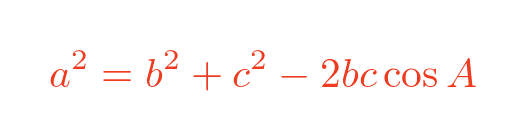## Finding a side using the cosine rule

In this example, we know the sides b and c, plus the enclosed angle A, and we wish to find the other side, a.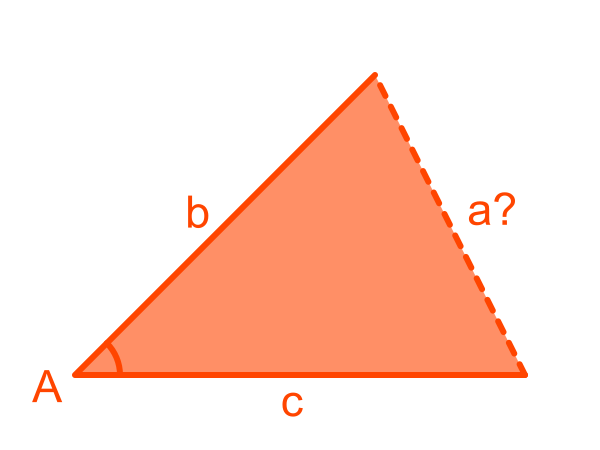The cosine rule lets us find a squared, so to find a we need to take the square root of both sides. Here is the formula: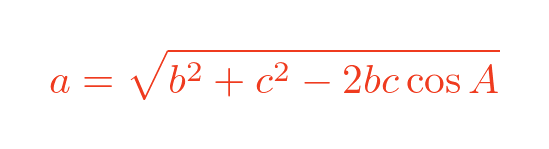## Finding an angle using the cosine rule

In this example we know the sides a, b and c, so we can find the angle, A: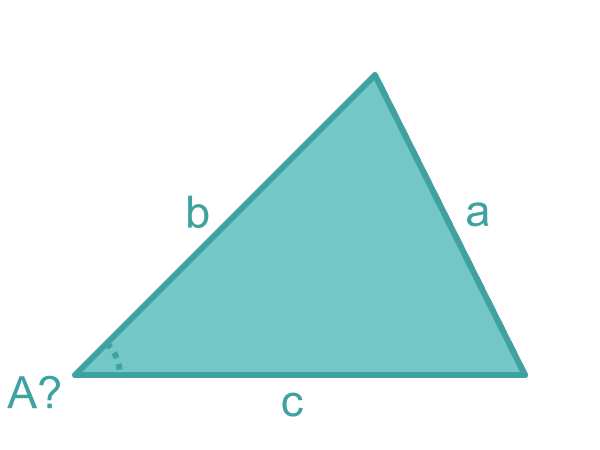The cosine rule can be rearranged to find the cosine of A. Here is the formula: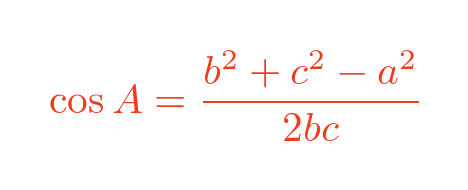In both cases, we will know the value of one angle and all three sides. We can then use the sine rule to find the other angles.